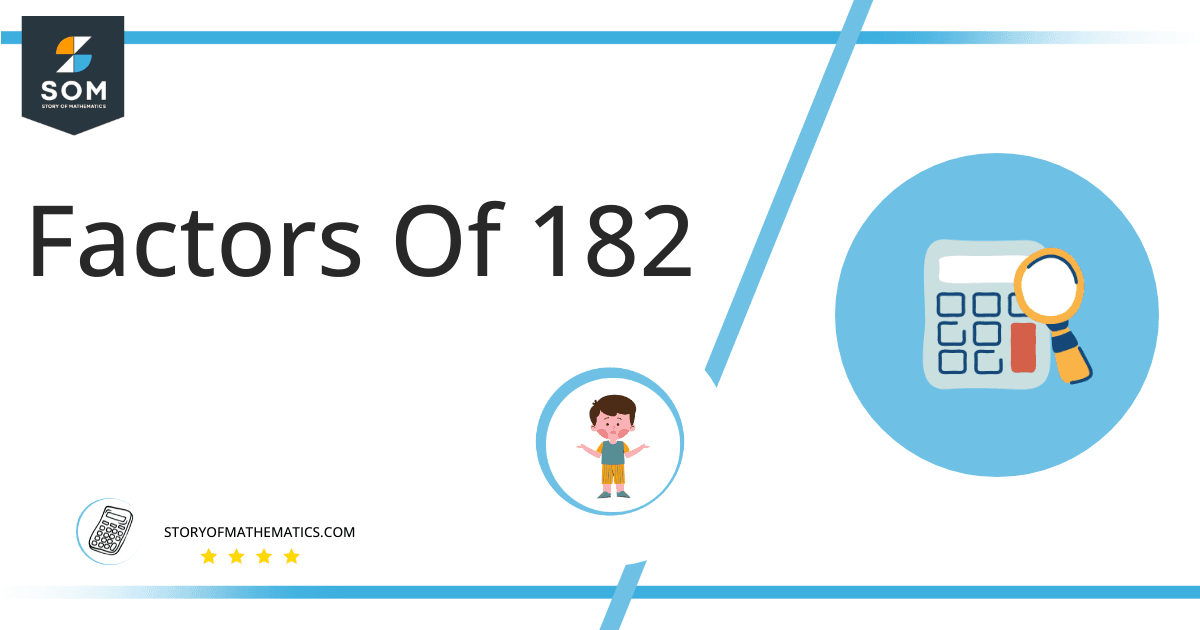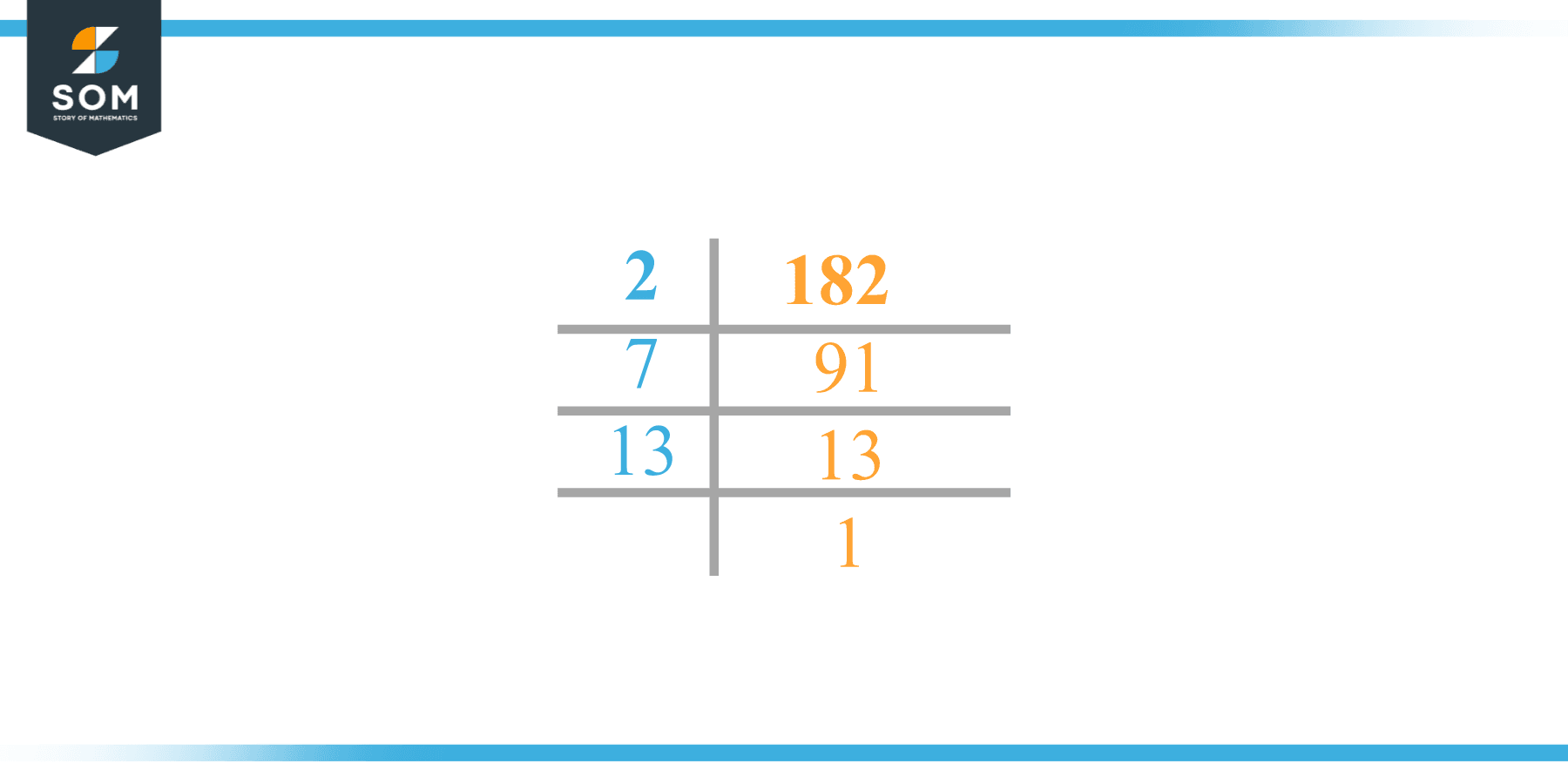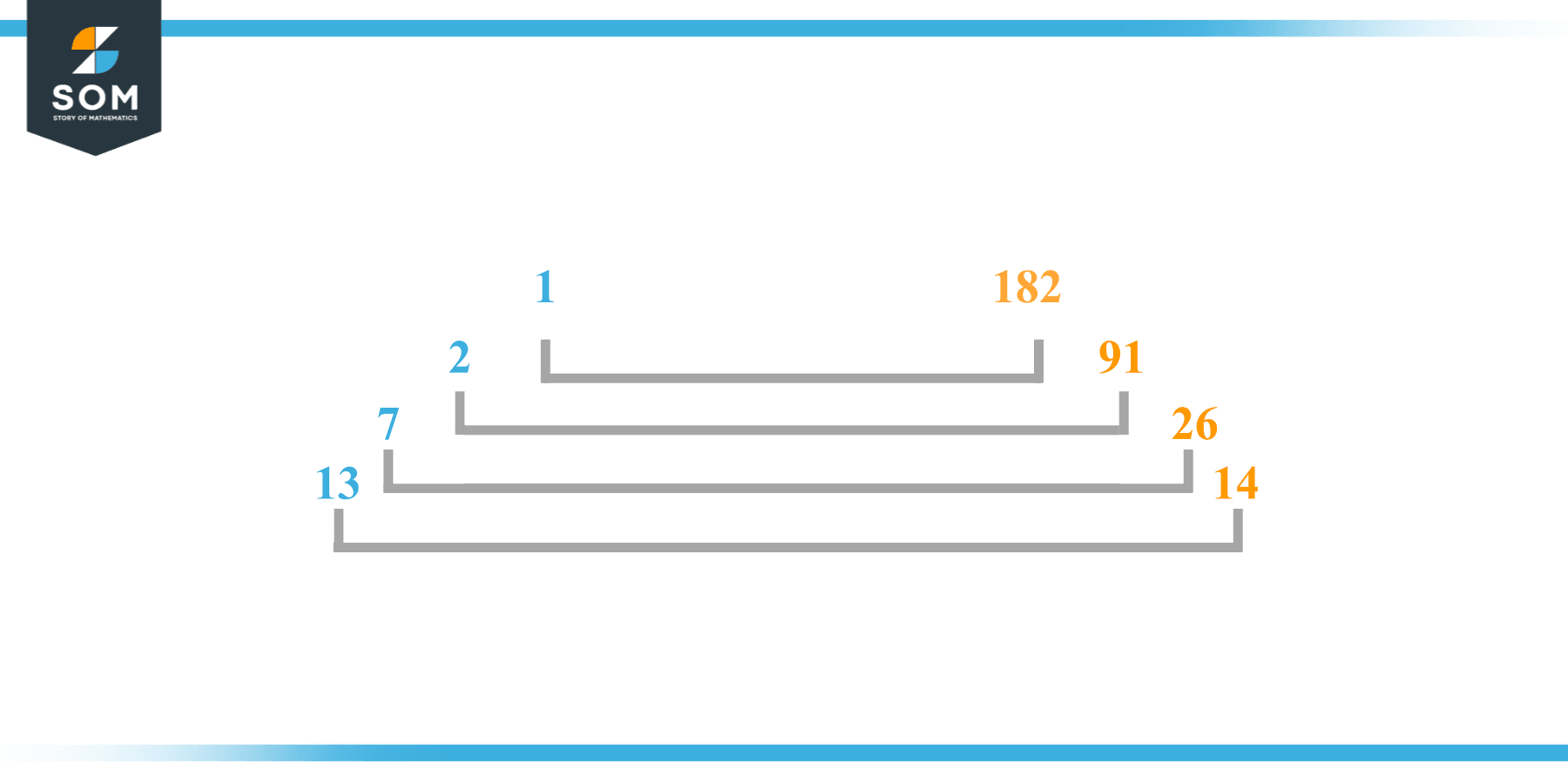# Factors of 182: Prime Factorization, Methods, and Examples

The number182 is an even composite number. It has 8 factors in total. All the numbers are called 182’s factors as they do not leave any remainder upon division by 182.The factors of the given number can be positive as well as negative provided that the given number is achieved upon multiplication of two-factor integers.

### Factors of 182

Here are the factors of number 182.

Factors of 182: 1, 2, 7, 13, 14, 26, 91, 182

### Negative Factors of 182

The negative factors of 182 are similar to its positive factors, just with a negative sign.

Negative Factors of 182: -1, -2, -7, -13, -14, -26, -91, -182

### Prime Factorization of 182

The prime factorization of 182 is the way of expressing its prime factors in the product form.

Prime Factorization: 2 x 7 x 13

In this article, we will learn about the factors of 182 and how to find them using various techniques such as upside-down division, prime factorization, and factor tree.

## What Are the Factors of 182?

The factors of 182 are1, 2, 7, 13, 14, 26, 91, and 182 . All of these numbers are the factors as they do not leave any remainder when divided by 182.

The factors of 182 are classified as prime numbers and composite numbers. The prime factors of the number 182 can be determined using the technique of prime factorization.

## How To Find the Factors of 182?

You can find the factors of 182 by using the rules of divisibility. The divisibility rule states that any number, when divided by any other natural number, is said to be divisible by the number if the quotient is the whole number and the resulting remainder is zero.

To find the factors of 182, create a list containing the numbers that are exactly divisible by 182 with zero remainders. One important thing to note is that 1 and 182 are the 182’s factors as every natural number has 1 and the number itself as its factor.

1 is also called the universal factor of every number. The factors of 182 are determined as follows:

$\dfrac{182}{1} = 182$

$\dfrac{182}{2} = 91$

$\dfrac{182}{7} = 26$

$\dfrac{182}{13} = 14$

$\dfrac{182}{182} = 1$

Therefore, 1, 2, 7, 13, 14, 26, 91, and 182 are the factors of 182.

### Total Number of Factors of 182

For 182 there are 8 positive factors and 8 negative ones. So in total, there are 16 factors of 182.

To find the total number of factors of the given number, follow the procedure mentioned below:

1. Find the factorization of the given number.
2. Demonstrate the prime factorization of the number in the form of exponent form.
3. Add 1 to each of the exponents of the prime factor.
4. Now, multiply the resulting exponents together. This obtained product is equivalent to the total number of factors of the given number.

By following this procedure the total number of factors of 182 is given as:

Factorization of X is 1 x 2 x 7 x 13.

The exponent of 1, 2, 7, and 13 is 1.

Adding 1 to each and multiplying them together results in 16.

Therefore, the total number of factors of 182 is 16. 8 are positive and 8 factors are negative.

### Important Notes

Here are some important points that must be considered while finding the factors of any given number:

• The factor of any given number must be a whole number.
• The factors of the number cannot be in the form of decimals or fractions.
• Factors can be positive as well as negative.
• Negative factors are the additive inverse of the positive factors of a given number.
• The factor of a number cannot be greater than that number.
• Every even number has 2 as its prime factor, the smallest prime factor.

## Factors of 182 by Prime FactorizationThe number 182 is a composite. Prime factorization is a useful technique for finding the number’s prime factors and expressing the number as the product of its prime factors.

Before finding the factors of 182 using prime factorization, let us find out what prime factors are. Prime factors are the factors of any given number that are only divisible by 1 and themselves.

To start the prime factorization of 182, start dividing by its smallest prime factor. First, determine that the given number is either even or odd. If it is an even number, then 2 will be the smallest prime factor.

Continue splitting the quotient obtained until 1 is received as the quotient. The prime factorization of 182 can be expressed as:

182 = 2 x 7 x 13

## Factors of 182 in PairsThe factor pairs are the duplet of numbers that when multiplied together result in the factorized number. Factor pairs can be more than one depending on the total number of factors of the given numbers.

For 182, the factor pairs can be found as:

1 x 182 = 182

2 x 91 = 182

7 x 26 = 182

13 x 14 = 182

The possible factor pairs of 182 are given as (1, 182), (2, 91), (7, 26), and (13, 14 ).

All these numbers in pairs, when multiplied, give 182 as the product.

The negative factor pairs of 182 are given as:

-1 x -182 = 182

-2 x -91 = 182

-7 x -26 = 182

-13 x -14 = 182

It is important to note that in negative factor pairs, the minus sign has been multiplied by the minus sign due to which the resulting product is the original positive number. Therefore, -1, -2, -7, -13, -14, -26, -91, and -182 are called negative factors of 182.

The list of all the factors of 182 including positive as well as negative numbers is given below.

Factor list of 182: 1, -1, 2, -2, 7, -7, 13, -13, 14, -14, 26, -26, 91, -91, 182 and -182

## Factors of 182 Solved Examples

To better understand the concept of factors, let’s solve some examples.

### Example 1

How many factors of 182 are there?

### Solution

The total number of Factors of 182 is 8.

Factors of 182 are 1, 2, 7, 13, 14, 26, 91, and 182.

### Example 2

Find the factors of 182 using prime factorization.

### Solution

The prime factorization of 182 is given as:

182 $\div$ 2 = 91

91 $\div$ 7 = 13

13 $\div$ 13 = 1

So the prime factorization of 182 can be written as:

2 x 7 x 13 = 182